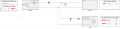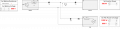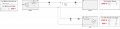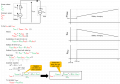# Calculation of DC link voltage and currents - Parallel battery / PSU / inverter

#### freeflyer

Joined Sep 9, 2016
13
I am going round in circles trying to understand how a battery (lithium) , PSU and inverter (traction) interact with each other by working out the voltages and currents.

Some notes:

- The PSU supplements the battery and will be used in 'power limit' mode.
- Any excess power from the PSU will be used to charge the battery AND power the inverter
- The PSU will have sense wires 'after' the series power diode (the power diode is to prevent current feeding back into the PSU).

I have created an interactive Excel spreadsheet, but I'm struggling with the equations to calculate the voltages and currents.

Screenshots are shown below for three different scenarios:

--------------------------------------------------------------------------------------------------------------------------------------------------------------------
1. PSU charges battery (inverter not running)

In this scenario:

- the inverter is not drawing any power (0 W)
- the battery open circuit voltage is 600 V
- the battery internal resistance is 1 R
- the PSU power limit is set to 1,000W
- the PSU voltage is set to 800 VQ. How do I calculate the DC link voltage ? My understanding is that the battery will 'clamp' the PSU voltage, so despite the PSU being set to 800 V the DC link will be clamped to just above 600 V.

Q. How do I calculate the PSU current (which will be the same as the battery current) ?

--------------------------------------------------------------------------------------------------------------------------------------------------------------------
2. PSU charges the battery AND powers the inverter

In this scenario:

- the inverter is drawing 500 W
- the battery open circuit voltage is 600 V
- the battery internal resistance is 1 R
- the PSU power limit is set to 1,000W
- the PSU voltage is set to 800 V
- the PSU supplies 500 W to the inverter, leaving an excess of 500 W to charge the batteryQ. How do I calculate the DC link voltage ? My understanding is that the battery will 'clamp' the PSU voltage, so despite the PSU being set to 800 V the DC link will be clamped to just above 600 V.

Q. How do I calculate the PSU current ?

Q. How do I calculate the battery current ?

Q. How do I calculate the inverter current ?
--------------------------------------------------------------------------------------------------------------------------------------------------------------------

3. PSU and battery power the inverter

In this scenario:

- the inverter is drawing 2,000 W
- the battery open circuit voltage is 600 V
- the battery internal resistance is 1 R
- the PSU power limit is set to 1,000 W
- the PSU voltage is set to 800 V
- the PSU supplies 1,000 W to the inverter and the battery supplies the remaining 1,000W to the inverterQ. How do I calculate the DC link voltage ? My understanding is that the battery will 'clamp' the PSU voltage, so despite the PSU being set to 800 V the DC link will be clamped to just above 600 V.

Q. How do I calculate the PSU current ?

Q. How do I calculate the battery current ?

Q. How do I calculate the inverter current ?
--------------------------------------------------------------------------------------------------------------------------------------------------------------------

The interactive spreadsheet is attached for reference 'PowerShareInteractive.zip' (I had to zip the spreadsheet as .xlsm files are prohibited) .

I would like to get this interactive spreadsheet working, but I don't fully understand how the circuit works and therefore the equations.

I had to use quadratic equations to try and solve the voltage and currents but I'm not sure this is correct, especially as the results are invalid in certain conditions.#### Attachments

• 181.2 KB Views: 0